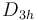# Problems with symmetry in VASP

Vasp can use the symmetry with ISYM = 2 for ordinaly GGA calculations, such as PBE. For hybrids ISYM = 3 is suggested.

VASP wiki tells that the following is symmetrized:

• The total charge density according to the determined space group
• The forces on the ions according to the determined space group.
• The stress tensor according to the determined space group

# Problems with SYMPREC

It looks beneficial lowering the symmetry precision to a level on which VASP identifies it. However if you look upwards, the geometry is not being symmetrized at all! Thus if you set SYMPREC = 0.1 as 0.1 angstroms, vasp will identify any symmetry with 0.1 error. However the geometry, and the resultant SCF cycle wont be running with any symmetry.

For example this a calculation of a distorted (by $v \infty$ symmetry, VASP identifies this system as$D_{3h}$ with SYMPREC = 0.1 setting. However the result never will be belong to the suggested symmetry, since only the forces and the charge density is symmetrized. By symmetrizing the forces the forces which should drive back the system into the high symmetry are being removed.